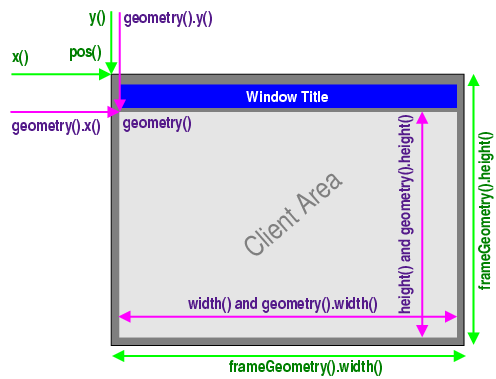1 2 3 4 5 6 7 8 9 10  class MyWindow(QtGui.QMainWindow): def __init__(self): QtGui.QMainWindow.__init__(self) desktop_geopmetry = QtGui.qApp.desktop().availableGeometry() self.move( desktop_geometry.width() - self.width(), desktop_geometry.height() - self.height() )1 2 3 4 5 6 7 8 9 10 11  class MyWindow(QtGui.QMainWindow): def __init__(self): QtGui.QMainWindow.__init__(self) desktop_geopmetry = QtGui.qApp.desktop().availableGeometry() self_geometry = self.frameGeometry() self.move( desktop_geometry.width() - self_geometry.width(), desktop_geometry.height() - self_geometry.height() ) 

  1 2 3 4 5 6 7 8 9 10 11 12 13 14 15 16 17  class MyWindow(QtGui.QMainWindow): def __init__(self): QtGui.QMainWindow.__init__(self) self.__first_show = True def showEvent(self, evt): evt.accept() if self.__first_show: desktop_geopmetry = QtGui.qApp.desktop().availableGeometry() self_geometry = self.frameGeometry() self.move( desktop_geometry.width() - self_geometry.width(), desktop_geometry.height() - self_geometry.height() ) self.__first_show = False